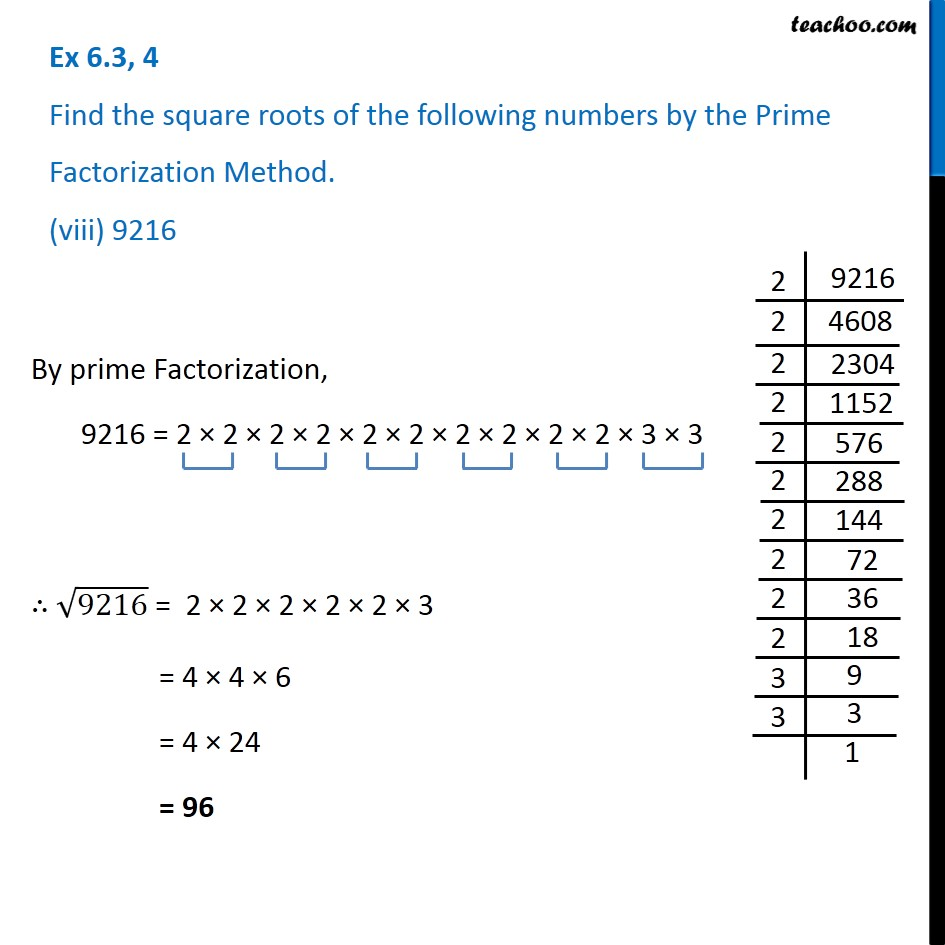1. Chapter 6 Class 8 Squares and Square Roots
2. Serial order wise
3. Ex 6.3

Transcript

Ex 6.3, 4 Find the square roots of the following numbers by the Prime Factorization Method. (viii) 9216 By prime Factorization, 9216 = 2 × 2 × 2 × 2 × 2 × 2 × 2 × 2 × 2 × 2 × 3 × 3 ∴ √9216 = 2 × 2 × 2 × 2 × 2 × 3 = 4 × 4 × 6 = 4 × 24 = 96

Ex 6.3

Chapter 6 Class 8 Squares and Square Roots
Serial order wise